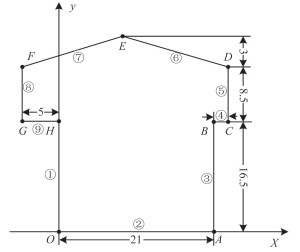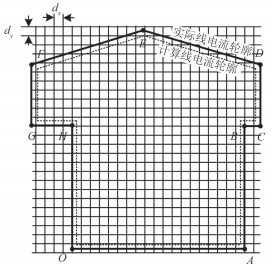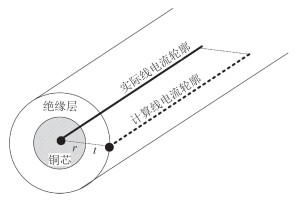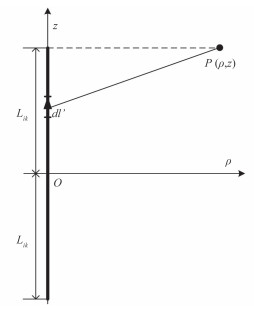﻿ 舰船消磁线圈的电感计算
 舰船科学技术2016, Vol. 38Issue (8): 100-103PDF

Inductance computation of ship degauss coil
SUN Ji, NI Shi-feng
Military Representative Office of Navy in Jiujiang Area, Jiujiang 332007, China
Abstract: Inductance of ship degaussing coil is an important engineering parameter, whose precise value is vital for designing degaussing coil and the peripheral circuit. For degaussing coil is special-shaped, while inductance equations those existing handbook provides are only fit for conventional geometric shape. Finite element method can deal with special shape with high precision, but mesh data is too large to implement for PC. Based on Biot-Savart principle, this paper proposes a numerical method for computing the inductance value of ship degaussing coil, discretizing the coil into several straight segments and areas enclosed into several rectangular elements. This method provides a new way for inductance computation of ship degaussing coil, and is very convenient to implement by computer program, promising to be extended to other specialshaped coil.
Key words: ship degauss     coil     inductance
1 舰船消磁线圈图 1 单匝消磁线圈示意图 Fig. 1 The schematic diagram of single-turn working coil
2 消磁线圈电感计算

2.1 经验公式计算法

 $L=\frac{\pi }{4}{{\mu }_{0}}{{w}^{2}}\frac{{{d}^{2}}}{a}{{K}_{\alpha }},$ (1)

 $L=\frac{\pi }{4}\times {{\mu }_{0}}\times {{86}^{2}}\times \frac{{{34}^{2}}}{200}\times 0.939143\approx 30.85\text{mH}\circ$ (2)
2.2 基于 Matlab 编程计算法

 $d\overset{\lower0.5em\hbox{$\smash{\scriptscriptstyle\rightharpoonup}$}} {B}=\frac{{{\mu }_{0}}}{4\pi }\frac{Id\overset{\lower0.5em\hbox{$\smash{\scriptscriptstyle\rightharpoonup}$}} {l}\times \overset{\lower0.5em\hbox{$\smash{\scriptscriptstyle\rightharpoonup}$}} {r}}{{{r}^{3}}},$ (3)图 2 简化后单一线圈所在坐标系示意图 Fig. 2 The simplified single-turn working coil in X-Y coordinate system

2.2.1 区域离散化

[X,Y] = meshgrid（-4.999∶0.011∶23,28∶ -0.011∶0）；% 生成 2 维平面网格，

Z1 =（Y <（3/14*（X + 5）+ 25））；% 第 7 段导体所在直线下方区域为逻辑 1，

Z2 =（Y <（-3/14*（X - 23）+ 25））；% 第 6 段导体所在直线下方区域为逻辑 1，

Z3 =（X > -4.984 9）；% 第 8 段导体所在直线右方区域为逻辑 1，

Z4 =（X < 22.984 9）；% 第 5 段导体所在直线左方区域为逻辑 1，

Z5 =（Y > 16.515 1）；% 线圈腰部上方区域为逻辑 1，

A1 = Z1 & Z2 & Z3 & Z4 & Z5；% 线圈内上半区域为逻辑 1，

Z6 =（X > 0.015 138 8）；% 第 1 段导体所在直线右方区域为逻辑 1，

Z7 =（X < 20.984 9）；% 第 3 段导体所在直线左方区域为逻辑 1，

Z8 =（Y > 0.015 138 8）；% 第 2 段导体所在直线下方区域为逻辑 1，

Z9 =（Y < 16.6）；% 线圈腰部下方区域为逻辑 1，

A2 = Z6 & Z7 & Z8 & Z9；% 线圈内下半区域为逻辑 1，

Z=A1|A2；% 上下半区域组成完整的线圈内部区域，即计算有效区域。图 3 线圈截面离散化 Fig. 3 The discretization of coil area

 $d=r+t,$ (4)图 4 线圈导线截面 Fig. 4 Cross section of wire

 $Z=5(i-1)\circ$ (5)
2.2.2 磁链计算

 \begin{align} & \underset{ik}{\mathop{{\overset{\lower0.5em\hbox{\smash{\scriptscriptstyle\rightharpoonup}$}} {B}}}}\,(\rho ,z)= \\ & \underset{\phi }{\mathop{{\overset{\lower0.5em\hbox{$\smash{\scriptscriptstyle\rightharpoonup}}} {e}}}}\,\frac{{{\mu }_{0}}{{N}_{i}}}{4\pi \rho }\left[ \frac{z+{{L}_{ik}}}{\sqrt{{{\rho }^{2}}+{{(z+{{L}_{ik}})}^{2}}}}-\frac{z-{{L}_{ik}}}{\sqrt{{{\rho }^{2}}+{{(z-{{L}_{ik}})}^{2}}}} \right]\circ \\ \end{align} (6)图 5 第 i 槽线圈槽第 k 段细导线通电产生的磁场 Fig. 5 Magnetic field generated by kth segment finite length straight wirein ith slot

i 槽线圈在空间产生的磁密为 9 段导体产生的磁密合成：

 $\underset{i}{\mathop{{\overset{\lower0.5em\hbox{$\smash{\scriptscriptstyle\rightharpoonup}$}} {B}}}}\,(\rho ,z)=\sum\limits_{k=1}^{9}{\underset{ik}{\mathop{{\overset{\lower0.5em\hbox{$\smash{\scriptscriptstyle\rightharpoonup}$}} {B}}}}\,(\rho ,z)},$ (7)

 ${{\phi }_{ij}}=\underset{i}{\mathop{{\overset{\lower0.5em\hbox{$\smash{\scriptscriptstyle\rightharpoonup}$}} {B}}}}\,({{\rho }_{j}},{{z}_{j}})\cdot \underset{j}{\mathop{{\overset{\lower0.5em\hbox{$\smash{\scriptscriptstyle\rightharpoonup}$}} {S}}}}\,{{N}_{j}},$ (8)

 $L=\psi =\sum\limits_{i=1}^{41}{\sum\limits_{j=1}^{41}{{{\phi }_{ij}}}}\circ$ (9)

3 结语

  江立军. 舰船物理场特性监测与视情维护[J]. 舰船科学技术 , 2008, 30 (4) :93–97. JIANG Li-jun. Property monitoring and condition-based maintenance for ship physical field[J]. Ship Science and Technology , 2008, 30 (4) :93–97.  腾广汉, 康建华. 螺线管磁场强度的级数法计算[J]. 电工技术学报 , 1993, 8 (4) :36–40. TENG Guang-han, KANG Jian-hua. A fast calculating method for magnetic field of solenoid[J]. Transactions of china Electrotechnical Society , 1993, 8 (4) :36–40.  吴素文. 空心圆柱线圈的电感计算表[J]. 郑州大学学报(工学版) , 2003, 24 (3) :106–112. WU Su-wen. Inductance tables of air-cored cylindrical coil[J]. Journal of Zhengzhou University (Engineering Science) , 2003, 24 (3) :106–112.  李新文, 松林, 恩克. 单匝线圈电感的计算[J]. 内蒙古师大学报自然科学(汉文)版 , 1999, 28 (1) :27–30. LI Xin-wen, SONG Lin, EN ke. The calculation of single-turn coil inductance[J]. Journal of Inner Mongolia Normal University (Natural Science Edition) , 1999, 28 (1) :27–30.  卡兰塔罗夫 П Л, 采伊特林 Л А.电感计算手册[M]. 陈汤铭, 刘保安, 罗应立, 等, 译. 北京: 机械工业出版社, 1992: 252-256, 387-390.  GROVER F W. Inductance calculation[M]. New York: Van-Nostrand, 1949 : 55 -60.  MILLER T J E, MCGILP M I, STATON D A, et al. Calculation of inductance in permanent-magnet DC motors[J]. IEE Proceedings-electric Power Applications , 1999, 146 (2) :129–137. DOI:10.1049/ip-epa:19990297